🎁 力扣圈子「你来写，我来送」优质内容征集活动• 积极创造优质原创内容
• 发起精彩讨论话题
• 参与讨论发表优质回答

————————————————— 以下是获奖用户 —————————————————

#### 10 月 11 日第八期获奖公布

🏆2019 力扣杯 - 全国秋季编程大赛

🏆第 157 场力扣周赛

LeetCode155 题解

「第 151 场周赛」题解

#### 8 月 16 日第一期获奖公布

「圈子」每期优质创作者将根据内容阅读量、内容质量、点赞数、活跃度等因素综合评定。

10 期「圈子」优质创作者评选活动已经结束，感谢所有用户为「圈子」贡献优质内容，同时也非常欢迎新同学创作更多优质内容，力扣君会不定期给优秀创作者发放福利，敬请期待～

19

6

• 积极创造优质原创内容，那么贴上对应的原创内容链接
• 发起精彩讨论话题，贴上对应的话题链接
• 参与讨论发表优质回答，贴上对应的截图之类的

7

3
4

1.直接插入排序

1. 将第一个数和第二个数排序，然后构成一个有序序列
2. 将第三个数插入进去，构成一个新的有序序列。
3. 对第四个数、第五个数……直到最后一个数，重复第二步。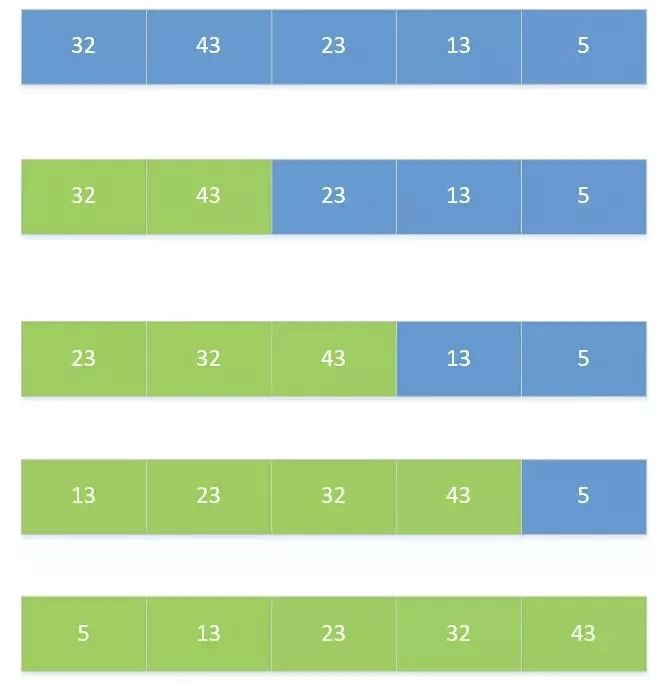1. 首先设定插入次数，即循环次数，for(int i=1;i<length;i++)，1个数的那次不用插入。
2. 设定插入数和得到已经排好序列的最后一个数的位数。insertNum和j=i-1。
3. 从最后一个数开始向前循环，如果插入数小于当前数，就将当前数向后移动一位。
4. 将当前数放置到空着的位置，即j+1。
代码实现如下：
publicvoidinsertSort(int[] a){
int length=a.length;//数组长度，将这个提取出来是为了提高速度。
int insertNum;//要插入的数
for(int i=1;i<length;i++){//插入的次数
insertNum=a[i];//要插入的数
int j=i-1;//已经排序好的序列元素个数
while(j>=0&&a[j]>insertNum){//序列从后到前循环，将大于insertNum的数向后移动一格
a[j+1]=a[j];//元素移动一格
j--;
}
a[j+1]=insertNum;//将需要插入的数放在要插入的位置。
}
}


2.希尔排序

1. 将数的个数设为n，取奇数k=n/2，将下标差值为k的书分为一组，构成有序序列。
2. 再取k=k/2 ，将下标差值为k的书分为一组，构成有序序列。
3. 重复第二步，直到k=1执行简单插入排序。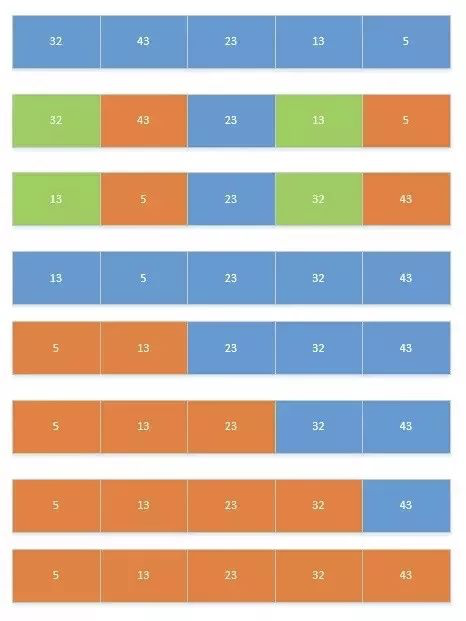1. 首先确定分的组数。
2. 然后对组中元素进行插入排序。
3. 然后将length/2，重复1,2步，直到length=0为止。
代码实现如下：
publicvoidsheelSort(int[] a){
int d = a.length;
while (d!=0) {
d=d/2;
for (int x = 0; x < d; x++) {//分的组数
for (int i = x + d; i < a.length; i += d) {//组中的元素，从第二个数开始
int j = i - d;//j为有序序列最后一位的位数
int temp = a[i];//要插入的元素
for (; j >= 0 && temp < a[j]; j -= d) {//从后往前遍历。
a[j + d] = a[j];//向后移动d位
}
a[j + d] = temp;
}
}
}
}


3.简单选择排序

(如果每次比较都交换，那么就是交换排序；如果每次比较完一个循环再交换，就是简单选择排序。)

1. 遍历整个序列，将最小的数放在最前面。
2. 遍历剩下的序列，将最小的数放在最前面。
3. 重复第二步，直到只剩下一个数。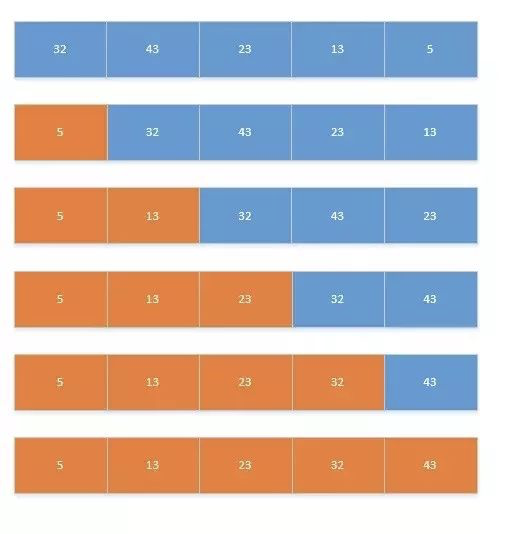1. 首先确定循环次数，并且记住当前数字和当前位置。
2. 将当前位置后面所有的数与当前数字进行对比，小数赋值给key，并记住小数的位置。
3. 比对完成后，将最小的值与第一个数的值交换。
4. 重复2、3步。
代码实现如下：
publicvoidselectSort(int[] a){
int length = a.length;
for (int i = 0; i < length; i++) {//循环次数
int key = a[i];
int position=i;
for (int j = i + 1; j < length; j++) {//选出最小的值和位置
if (a[j] < key) {
key = a[j];
position = j;
}
}
a[position]=a[i];//交换位置
a[i]=key;
}
}


4.堆排序

1. 将序列构建成大顶堆。
2. 将根节点与最后一个节点交换，然后断开最后一个节点。
3. 重复第一、二步，直到所有节点断开。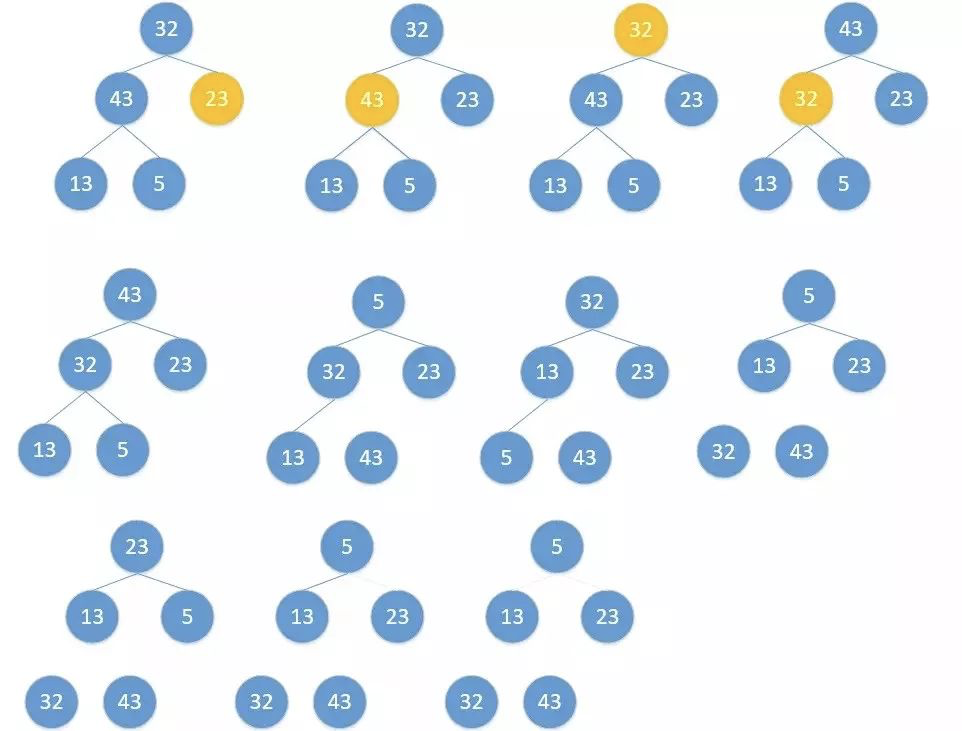publicvoidheapSort(int[] a){
System.out.println("开始排序");
int arrayLength=a.length;
//循环建堆
for(int i=0;i<arrayLength-1;i++){
//建堆
buildMaxHeap(a,arrayLength-1-i);
//交换堆顶和最后一个元素
swap(a,0,arrayLength-1-i);
System.out.println(Arrays.toString(a));
}
}
privatevoidswap(int[] data, int i, int j){
// TODO Auto-generated method stub
int tmp=data[i];
data[i]=data[j];
data[j]=tmp;
}
//对data数组从0到lastIndex建大顶堆
privatevoidbuildMaxHeap(int[] data, int lastIndex){
// TODO Auto-generated method stub
//从lastIndex处节点（最后一个节点）的父节点开始
for(int i=(lastIndex-1)/2;i>=0;i--){
//k保存正在判断的节点
int k=i;
//如果当前k节点的子节点存在
while(k*2+1<=lastIndex){
//k节点的左子节点的索引
int biggerIndex=2*k+1;
//如果biggerIndex小于lastIndex，即biggerIndex+1代表的k节点的右子节点存在
if(biggerIndex<lastIndex){
//若果右子节点的值较大
if(data[biggerIndex]<data[biggerIndex+1]){
//biggerIndex总是记录较大子节点的索引
biggerIndex++;
}
}
//如果k节点的值小于其较大的子节点的值
if(data[k]<data[biggerIndex]){
//交换他们
swap(data,k,biggerIndex);
//将biggerIndex赋予k，开始while循环的下一次循环，重新保证k节点的值大于其左右子节点的值
k=biggerIndex;
}else{
break;
}
}
}
}


5.冒泡排序

1. 将序列中所有元素两两比较，将最大的放在最后面。
2. 将剩余序列中所有元素两两比较，将最大的放在最后面。
3. 重复第二步，直到只剩下一个数。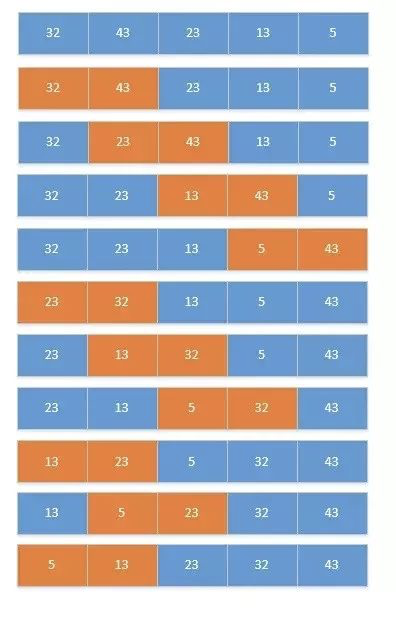1. 设置循环次数。
2. 设置开始比较的位数，和结束的位数。
3. 两两比较，将最小的放到前面去。
4. 重复2、3步，直到循环次数完毕。
代码实现如下：
publicvoidbubbleSort(int[] a){
int length=a.length;
int temp;
for(int i=0;i<a.length;i++){
for(int j=0;j<a.length-i-1;j++){
if(a[j]>a[j+1]){
temp=a[j];
a[j]=a[j+1];
a[j+1]=temp;
}
}
}
}


6.快速排序

1. 选择第一个数为p，小于p的数放在左边，大于p的数放在右边。
2. 递归的将p左边和右边的数都按照第一步进行，直到不能递归。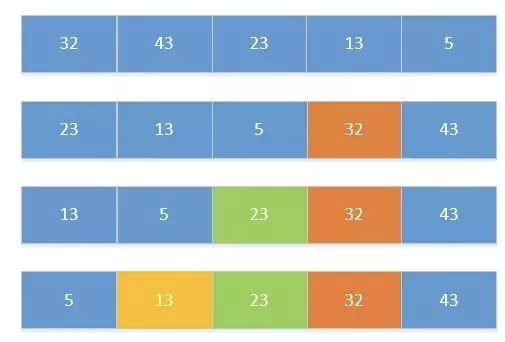publicstaticvoidquickSort(int[] numbers, int start, int end){
if (start < end) {
int base = numbers[start]; // 选定的基准值（第一个数值作为基准值）
int temp; // 记录临时中间值
int i = start, j = end;
do {
while ((numbers[i] < base) && (i < end))
i++;
while ((numbers[j] > base) && (j > start))
j--;
if (i <= j) {
temp = numbers[i];
numbers[i] = numbers[j];
numbers[j] = temp;
i++;
j--;
}
} while (i <= j);
if (start < j)
quickSort(numbers, start, j);
if (end > i)
quickSort(numbers, i, end);
}
}


7.归并排序

1. 选择相邻两个数组成一个有序序列。
2. 选择相邻的两个有序序列组成一个有序序列。
3. 重复第二步，直到全部组成一个有序序列。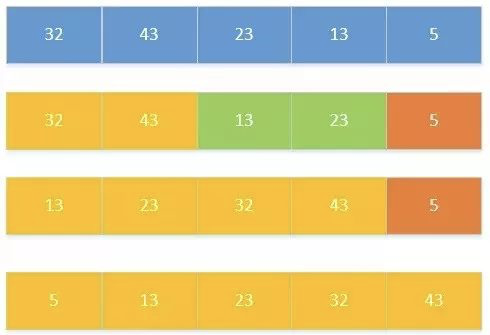publicstaticvoidmergeSort(int[] numbers, int left, int right){
int t = 1;// 每组元素个数
int size = right - left + 1;
while (t < size) {
int s = t;// 本次循环每组元素个数
t = 2 * s;
int i = left;
while (i + (t - 1) < size) {
merge(numbers, i, i + (s - 1), i + (t - 1));
i += t;
}
if (i + (s - 1) < right)
merge(numbers, i, i + (s - 1), right);
}
}
privatestaticvoidmerge(int[] data, int p, int q, int r){
int[] B = newint[data.length];
int s = p;
int t = q + 1;
int k = p;
while (s <= q && t <= r) {
if (data[s] <= data[t]) {
B[k] = data[s];
s++;
} else {
B[k] = data[t];
t++;
}
k++;
}
if (s == q + 1)
B[k++] = data[t++];
else
B[k++] = data[s++];
for (int i = p; i <= r; i++)
data[i] = B[i];
}


8.基数排序

1. 将所有的数的个位数取出，按照个位数进行排序，构成一个序列。
2. 将新构成的所有的数的十位数取出，按照十位数进行排序，构成一个序列。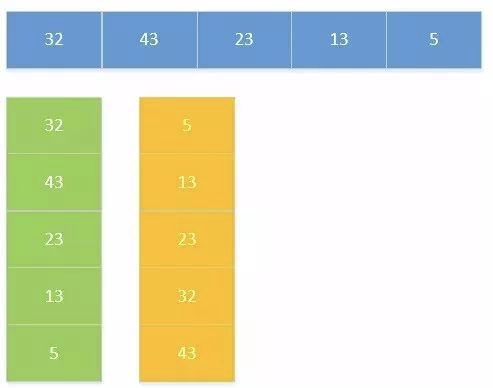publicvoidsort(int[] array){
//首先确定排序的趟数;
int max = array;
for (int i = 1; i < array.length; i++) {
if (array[i] > max) {
max = array[i];
}
}
int time = 0;
//判断位数;
while (max > 0) {
max /= 10;
time++;
}
//建立10个队列;
List<ArrayList> queue = new ArrayList<ArrayList>();
for (int i = 0; i < 10; i++) {
ArrayList<Integer> queue1 = new ArrayList<Integer>();
}
//进行time次分配和收集;
for (int i = 0; i < time; i++) {
//分配数组元素;
for (int j = 0; j < array.length; j++) {
//得到数字的第time+1位数;
int x = array[j] % (int) Math.pow(10, i + 1) / (int) Math.pow(10, i);
ArrayList<Integer> queue2 = queue.get(x);
queue.set(x, queue2);
}
int count = 0;//元素计数器;
//收集队列元素;
for (int k = 0; k < 10; k++) {
while (queue.get(k).size() > 0) {
ArrayList<Integer> queue3 = queue.get(k);
array[count] = queue3.get(0);
queue3.remove(0);
count++;
}
}
}
}

2
1

app 的好像不能分类别，是不是

1
1

1